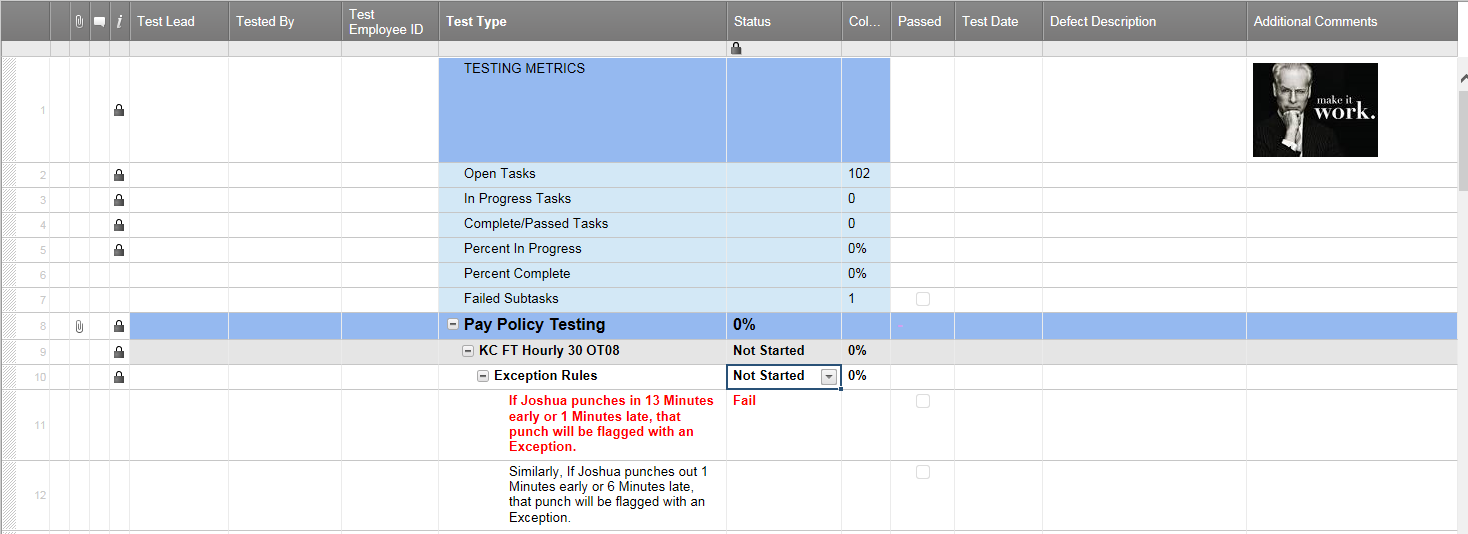#### Welcome to the Smartsheet Forum Archives

The posts in this forum are no longer monitored for accuracy and their content may no longer be current. If there's a discussion here that interests you and you'd like to find (or create) a more current version, please Visit the Current Forums.

# Need help with a formula

here are the formulas and a look at the Spreadhseet.

The Column labeled Column has the following formula

=SUM((COUNTIF(CHILDREN(Passed10), 1) / SUM(COUNT(CHILDREN(Passed10)))))

The current formula in the Status column for the Exception Rules row10 is =IF(Column10 = 1, "Pass", IF(Column10 = 0, "Not Started", IF(Column10 <> 1, "Review In Progress"))) which works unless I have a task with only 1 subtask and that subtask fails....

I tried variations of =IF(CHILDREN(Status10)= "Fail","Failed Subtask", IF(Column16 = 1, "Pass", IF(Column16 <> 1, "Review In Progress", "Not Started")))) but keep getting the dreaded Unparseable error...

I also tried =IF (OR(CHILDREN(Status10) = "Fail", (Column10) <> 1), "Review in Progress", IF((Column10) = 1, "Pass", "Not Started")))

The desired outcome is for the Status on row 10 to reflect Failed Subtask when Fail is in the Children of Status10, or if the total in Column10 is not equal to 1 I want to see Review in Progress, if the total in Column10 equals 1 I want to see Pass...

Any ideas on what I need to do to make this work?Tags: